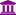Member Posts: 2Contributor I
edited September 2019 in Help

Hi,

I m a student from Pakistan. I am not much familiar with Rapidminer. I am given a task of market basket analysis and have almost 10,000 rows of data to apply FP-growth and apriori.

My given data is in the format:

2 milk cake

3 cake, cheese, milk

and for apriori algorithm I need to convert data into binary matrix format like:

TID | cheese bread milk cake

1 | 1 1 1 0

2| 0 0 1 1

3| 1 0 1 1

how can I preprocess my data in rapidminer to get this format

thanks in advanceTagged:

• Moderator, RapidMiner Certified Analyst, Member Posts: 1,195Unicorn

Hi @RobotGirl,

For the moment, I don't know how to perform your data transformation with RapidMiner's native operators.(I will think about it)

So waiting, I propose a Python script :

I assume that your initial dataset is under this form :By executing the process, you obtain a dataset like that :the process :

`<?xml version="1.0" encoding="UTF-8"?><process version="9.0.001">  <context>    <input/>    <output/>    <macros/>  </context>  <operator activated="true" class="process" compatibility="9.0.001" expanded="true" name="Process">    <process expanded="true">      <operator activated="true" class="read_excel" compatibility="9.0.001" expanded="true" height="68" name="Read Excel" width="90" x="112" y="34">        <parameter key="excel_file" value="C:\Users\Lionel\Documents\Formations_DataScience\Rapidminer\Tests_Rapidminer\Data_Preparation\Purchases_2.xlsx"/>        <list key="annotations">          <parameter key="0" value="Name"/>        </list>        <parameter key="date_format" value="MMM d, yyyy h:mm:ss a z"/>        <list key="data_set_meta_data_information">          <parameter key="0" value="Id.true.integer.attribute"/>          <parameter key="1" value="B.true.polynominal.attribute"/>          <parameter key="2" value="C.true.polynominal.attribute"/>          <parameter key="3" value="D.true.polynominal.attribute"/>        </list>        <parameter key="read_not_matching_values_as_missings" value="false"/>      </operator>      <operator activated="true" class="select_attributes" compatibility="9.0.001" expanded="true" height="82" name="Select Attributes" width="90" x="246" y="34">        <parameter key="attribute_filter_type" value="single"/>        <parameter key="attribute" value="Id"/>        <parameter key="invert_selection" value="true"/>      </operator>      <operator activated="true" class="python_scripting:execute_python" compatibility="7.4.000" expanded="true" height="82" name="Execute Python" width="90" x="380" y="34">        <parameter key="script" value="import pandas as pd&#10;import numpy as np&#10;&#10;# rm_main is a mandatory function, &#10;# the number of arguments has to be the number of input ports (can be none)&#10;&#10;&#10;def rm_main(data):&#10;&#10;  def get_series(string): &#10;    return (data == string).T.any() &#10;&#10;  cols = np.unique(data.stack().values).tolist() &#10;  data_2 = pd.DataFrame(columns=cols, index=range(len(data))) &#10;  &#10;  for col in cols: &#10;    data_2[col] = get_series(col)&#10;&#10;    &#10;  return data_2"/>      </operator>      <operator activated="true" class="generate_id" compatibility="9.0.001" expanded="true" height="82" name="Generate ID" width="90" x="514" y="34"/>      <connect from_op="Read Excel" from_port="output" to_op="Select Attributes" to_port="example set input"/>      <connect from_op="Select Attributes" from_port="example set output" to_op="Execute Python" to_port="input 1"/>      <connect from_op="Execute Python" from_port="output 1" to_op="Generate ID" to_port="example set input"/>      <connect from_op="Generate ID" from_port="example set output" to_port="result 1"/>      <portSpacing port="source_input 1" spacing="0"/>      <portSpacing port="sink_result 1" spacing="0"/>      <portSpacing port="sink_result 2" spacing="0"/>    </process>  </operator></process>`

To execute this process you need :

- to install Python on your computer

- to install Execute Python operator (from the marketPlace)

I hope it helps,

Regards,

Lionel

• Member Posts: 2Contributor I

thanks for your respond @lionelderkrikor  but my task is to use a rapidminer tool without any external coding.

• Member Posts: 270Unicorn

You can do it directly with the new version of the FP-Growth operator.

Your dataset (CSV file should be like this):

2;milk,cake
3;cake,cheese,milk

Please notice the ';'. This is the column separator. So this dataset has only two colums 'id' and 'basket'.

Read it into your repository. It should look like the image below:Set the first column to the role of ID.

When you use the FP-growth operator make sure that in Input format you select 'items list in a column' and the item separators is set to  ','.

Now run the process below:

`<?xml version="1.0" encoding="UTF-8"?><process version="9.0.001">  <context>    <input/>    <output/>    <macros/>  </context>  <operator activated="true" class="process" compatibility="9.0.001" expanded="true" name="Process">    <process expanded="true">      <operator activated="true" class="retrieve" compatibility="9.0.001" expanded="true" height="68" name="Retrieve fpgrowth" width="90" x="112" y="85">        <parameter key="repository_entry" value="//Clases/fpgrowth"/>      </operator>      <operator activated="true" class="set_role" compatibility="9.0.001" expanded="true" height="82" name="Set Role" width="90" x="313" y="85">        <parameter key="attribute_name" value="id"/>        <parameter key="target_role" value="id"/>        <list key="set_additional_roles"/>      </operator>      <operator activated="true" class="concurrency:fp_growth" compatibility="9.0.001" expanded="true" height="82" name="FP-Growth" width="90" x="514" y="85">        <parameter key="input_format" value="item list in a column"/>        <parameter key="item_separators" value=","/>        <parameter key="min_support" value="0.01"/>        <enumeration key="must_contain_list"/>      </operator>      <connect from_op="Retrieve fpgrowth" from_port="output" to_op="Set Role" to_port="example set input"/>      <connect from_op="Set Role" from_port="example set output" to_op="FP-Growth" to_port="example set"/>      <connect from_op="FP-Growth" from_port="example set" to_port="result 1"/>      <connect from_op="FP-Growth" from_port="frequent sets" to_port="result 2"/>      <portSpacing port="source_input 1" spacing="0"/>      <portSpacing port="sink_result 1" spacing="0"/>      <portSpacing port="sink_result 2" spacing="0"/>      <portSpacing port="sink_result 3" spacing="0"/>    </process>  </operator></process>`

• Member, University Professor Posts: 7University Professor

How would you preprocess the original CSV to replace the first ',' with a ';'?

A few minutes later of googling the answer:

1) Open the CSV in any decent editor (atom,ultraedit,notepad++,etc)

2) Find:

`^([^,]*),`

3) Replace

`\$1;`

• Administrator, Moderator, Employee, RapidMiner Certified Analyst, RapidMiner Certified Expert, University Professor Posts: 3,453RM Data Scientist

and you don't need to use a editor but can use rapidminer's Replace operator for itCheers,

Martin

- Sr. Director Data Solutions, Altair RapidMiner -
Dortmund, Germany